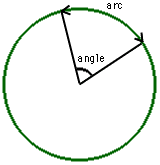Date: Thu, 5 Feb 1998 05:05:33 -0600 (CST)
Subject: The Earth's Arc

Name: Robert

Level: Secondary

Question:
How do I find the arc/mile of the earths surface? What is the arc?

Hi Robert,My reference book says that the equatorial radius of the earth is 3963 miles. Thus the circumference at the equator is 2*pi*r=24900 miles. The length of an arc on a circle is measured by the size of the angle at the center of the circle. Hence there are 360 degrees of arc for the entire circumference of 24900 miles or 360/24900=0.0145 degrees of arc per mile. I have more often seen the reciprocal of this number, that is 24900/360=69 miles per degree. If you look at a globe you will see that the lines of longitude are approximately 69 miles apart at the equator.
Cheers,
Harley

Go to Math Central